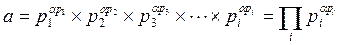資訊與網路安全技術：第三章 現代公開鑰匙系統      下一頁3-2 公開鑰匙的數學基礎

『數論』（Number Theory是公開鑰匙密碼學的理論基礎，本節就相關部份做簡單介紹，讀者若有興趣可參考。

『質數』（Prime是密碼系統主要計算數值，因為密碼系統大多採用『模數』（Modulo算術推論出來的。質數在『模數』運算裡出現的重複性最低，計算結果最能接近『唯一性』。

『質數』（Prime：一個只能被 1 或自己整除的數值，稱之為質數。質數是除了 1 和自己之外，除以小於本身的任何數都會有餘數，下列是1 100 之間的質數：

 2, 3, 5, 7, 11, 13, 17, 19, 23, 29, 31, 37, 41, 43, 47, 53, 59, 61, 67, 71, 73, 79, 83, 89, 973600 = 24× 32× 52

p2 = 3、α3 =2（因 p2 = 3，則αp2 =α3）；

p3 = 5、α5 =2（因 p3 = 5，則αp3 =α5）。

12 = 22× 31，則α2 =2、α3 =1

18 = 21× 32，則α2 =1、α3 =2

216 = 23× 33，因為α2 =2 + 1= 3、α3 =1 + 2 =3

gcd(a, b) 表示 a b 的最大公因數，則 gcd(a, b) = 1表示 a b 互質；也就是說，a b 之間除了 1 之外，沒有其他公因數。現在我們來觀察如何計算出兩數之間的最大公因數。首先將任意兩數分解出其對應的質因數冪次方的乘積，接著選取較低冪次方的因子，將這些因子相乘便是兩數的最大公因數。譬如，欲找出 18 300 兩數的最大公因數 gcd(18, 300)，可由下列計算式得到：

300 =  22× 31× 52

18 =  21× 32× 50，則：

gcd(300, 18) = 21× 31× 50 = 6